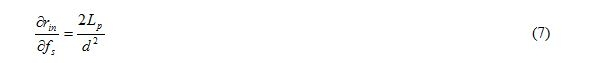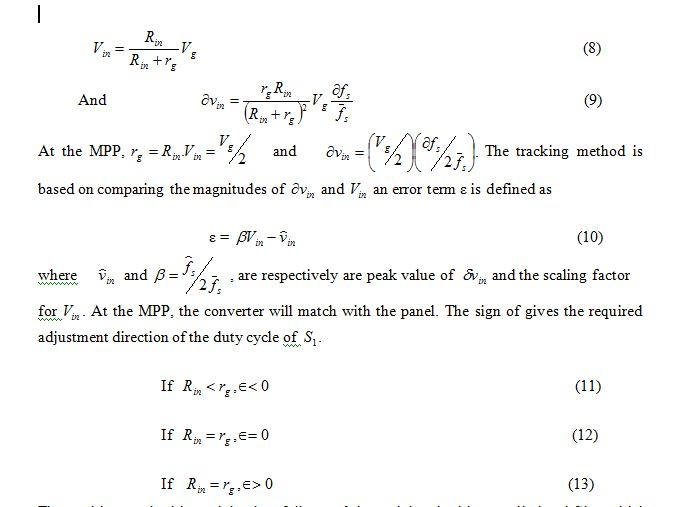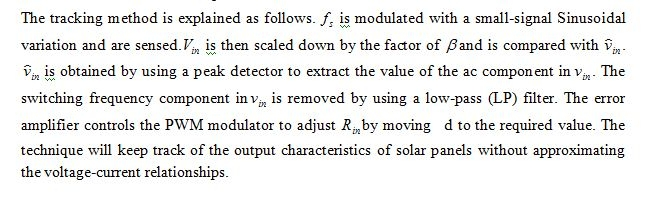Engineering

# Report on Maximum Power Point Tracking of the Panel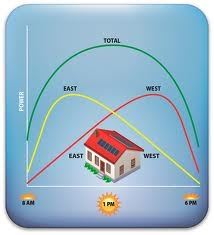TRACKING the maximum power point (MPP) of a photostatic (PV) array is usually an essential part of a PV system. As such, many MPP tracking (MPPT) methods have been developed and implemented. The methods vary in complexity, sensors required, convergence speed, cost, range of effectiveness, implementation hardware, popularity, and in other respects. They range from the almost obvious (but not necessarily ineffective) to the most creative (not necessarily most effective). In fact, so many methods have been developed that it has become difficult to adequately determine which method, newly proposed or existing, is most appropriate for a given PV system.

A maximum power point tracker (MPPT) is a high efficiency DC to DC converter that presents an optimal electrical load to a solar panel or array and produces a voltage suitable for the load. PV cells have a single operating point where the values of the current (I) and  (V) of the cell result in a maximum power output. These values correspond to a particular resistance, which is equal to V/I as specified by Ohm’s law. A PV cell has an exponential relationship between current and voltage, and the maximum power point (MPP) occurs at the knee of the curve, where the resistance is equal to the negative of the differential resistance (V/I = – dV / dI ). Maximum power point trackers utilize some type of control circuit or logic to search for this point and thus to allow the converter circuit to extract the maximum power available from a cell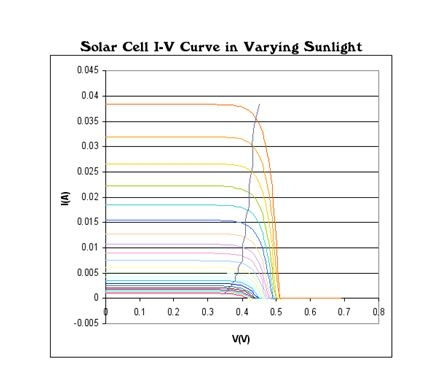Figure (28): Solar cell I-V curves where a line intersects the knee of the curves where the maximum power point is located

Tracking the maximum power point   (MPP) of the solar panel is based on the frequency modulation technique in  that the PWM signal to  is modulated with a low-frequency signal. The panel is modeled by a Thevenin’s equivalent circuit, which consists of a voltage source  connected in series in an output resistance  around the MPP. Both and  are subject to the level of insulation and temperature. The input voltage and equivalent Input resistance of the converter are  and  respectively. Assuming 100% conversion efficiency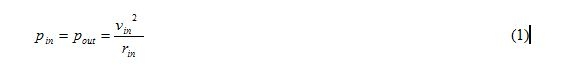The rate on change of  with respect to  and  can be shown to be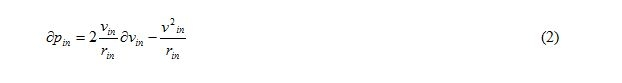At the MPP,  and .HenceWhere  is the average input voltage. This equation gives the required dynamic input

Characteristics of the converter, in which has small-signal variation of  subject to a small-signal change of  .

If the switching frequency  of   of is modulated with a small signal sinusoidal variation.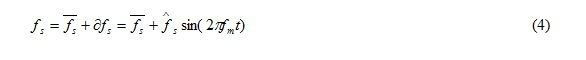Where  is the nominal switching frequency, is the modulating frequency and is much

lower than  ,  and  is the maximum frequency deviation.  Consists of two components, including an average resistance and    a small variation. That is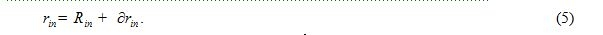As shown in , for the buck-boost converter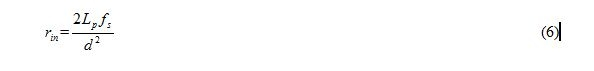By differentiating (7) it can be shown that…..